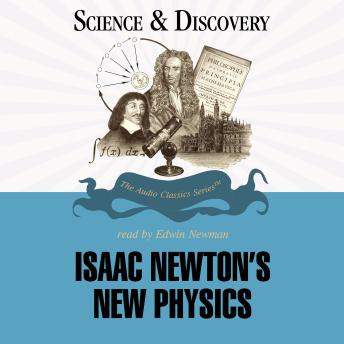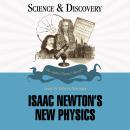# Isaac Newton's New Physics

#### Summary:

Isaac Newton (1642-1727) achieved momentous breakthroughs in three areas: mathematics (the calculus), a theory of colors, and gravitational attraction. His first insights in each of these areas occurred during the "wonder years" of 1665-66, when retreat from the plague isolated Newton at Woolsthorpe. Newton's theory of "fluxions", along with independent work by Leibniz, created the methods of modern calculus. Building on the analytic geometry of Rene' Descartes, these techniques allow us to reason about the infinitely small and infinitely small and infinitely large in a mathematically rigorous way. Newton also revised Descartes' theory of light to show that white light is composed of different rays, each associated with a specific angle of refraction and a specific color. Newton also invented the reflection telescope, and considered his work on light to be his first great success. The famous theory of gravitation was built on the foundation of Galileo's laws of terrestrial motion and Kepler's laws of celestial motion. Newton described the inverse-square law of gravitation (F=1/r2); his famous Principia of 1686 included three famous laws: 1. Bodies continue in motion or at rest unless changed by a force. 2. Force equals mass times acceleration (F=ma). 3. Every action has an equal and opposite reaction. Newton also provided the universal law of gravitation (F=Gm, m2/r2) and showed that the gravitational force (the factor G in this equation) is constant for all bodies.

## Isaac Newton's New Physics

#### by Dr. Gordon Britian

has successfully been added to your shopping cart